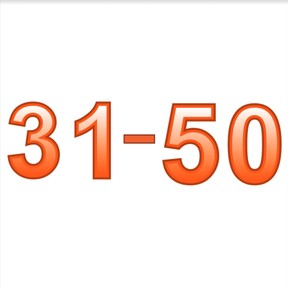Know numbers to 50

# Know numbers to 50

Know numbers to 50

No account needed.8,000 schools use Gynzy92,000 teachers use Gynzy1,600,000 students use Gynzy

## General

Students learn to recognize numbers 31 to 50 from their spoken form and can write down the numbers. Students can say the numbers, write the numbers, and write the numbers in words.

1.NBT.A.1

## Relevance

Discuss with students that numbers are everywhere and it is important to recognize them. An example is if you want to know and recognize someone's house number.

## Introduction

Students practice recognizing numbers to 30 by circling pairs of numbers.

## Development

The teacher points at the numbers 31 to 50 and says them aloud. Ask the students to repeat the number after you. Explain how to write numbers using the numbers 34 and 43 as examples. These numbers are easy to mix up while writing. Ask students to practice writing numbers. Say a number aloud and the students write it down. Check answers via the interactive whiteboard. Next, point at a number on the interactive whiteboard and ask students to say the number aloud.

Check that students have understood by asking the following questions:
Where might you see these numbers?
Can you give an example? (speed signs, bills, birthday cards)

## Guided Practice

Students are first asked to link a spoken number to a visual number. Next they are asked to link a visual number to a spoken number, and finally to note the number of a spoken number.

## Closing

Discuss with students that it is useful to recognize numbers to 50. Numbers are everywhere. An example is if you want to know the number of somebody's house. Check that students have understood by putting them in pairs and asking them to practice with number cards from 31 to 50. The fist student lays down a number and the second must say the number as quickly as possible. Take turns.

## Teaching Tip

Students who have difficulties can practice with saying and writing the numbers to 30. The students who need more challenge can start work on naming and writing numbers to 100.

## Instruction materials

Number cards from 31 to 50.

### The online teaching platform for interactive whiteboards and displays in schools

• Save time building lessons

• Manage the classroom more efficiently

• Increase student engagement